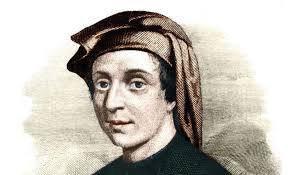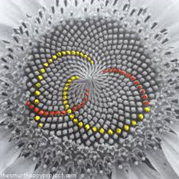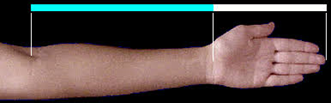# NCI BlogThe 23rd day of November is a special day for those among us that have a passion for number sequences. It has been named ‘Fibonacci Day’ after the 12th century mathematician Leonardo Pisano, a member of the Bonacci family. In particular, the naming of the day is due to the representation of the day in mm-dd calendar notation form, in this case 11-23, which represents the first four digits of the famed Fibonacci sequence.

The name Fibonacci has become synonymous with the Fibonacci sequence of numbers – a sequence of numbers where each number is the sum of the previous two numbers in the sequence; the sequence starts with the number 1, with the second sequence number also being a 1 the third number being 2 which is the sum of the first and second (1 + 1 = 2), continuing this process gives rise to the well-known infinite Fibonacci sequence of numbers: 1, 1, 2, 3, 5, 8, 13, ... However, Fibonacci is also recognised as one of the more accomplished mathematicians of his period.Some properties of the sequence concern the ratio of any sequence number to the previous number in the sequence, for example, 5 and 3, 5 divided by 3 being equal to 1.667 approximately, or even 13 and 8, 13 divided by 8 being equal to 1.625 approximately; or 6765 and 4181 – the 20th and 19th Fibonacci numbers, 6765 divided by 4181 being equal to 1.618 approximately. This ratio tending toward what is termed the ‘golden ratio,’ a ratio that appears to manifest itself in numerous natural places. For example, from the spirals appearing within sunflower seed flowers, to its appearance in human anatomy - representing the ratio of the length from the wrist to theelbow relative to the length of the hand, which equals approximately 1.618. The number of petals on a flower is a Fibonacci number. It has been said that Stradivarius used the ‘golden ratio’ in the design of his violins.Image: Bonnie Kittle

It is possible, that due to what seems like an infinite number of natural occurrences of the ‘golden ratio,’ which naturally derives from comparison of pairwise Fibonacci numbers has also added to the sequences mystical stature. Lending to this mystique also being the appearance of the Fibonacci concept in modern popular Hollywood blockbusters. The 2006 Dan Brown movie The Da Vinci Code certainly contributing to the elevation of the sequence to a mystical position in today’s culture. The sequence also receiving mention in the 2008 movie 21 and more recently in the 2016 movie Arrival. The paintings of Da Vinci and in particular his Last Supper, have numerous examples of the golden ratio. Maybe this can explain its appeal to conspiracy theorists, in that it can be found so naturally in nature and art, that it helps to put some rigour to their claims – who will dispute a theory supported by mathematics.

Modern uses of Fibonacci sequences can be found within the financial markets, with traders commonly using what has been termed ‘Fibonacci Retracement’ as a technical tool for the prediction of breakouts and breakdown points, also being used as buy triggers during uptrends. Facebook's new headquarters in Ballsbridge will have an address at 'Fibonacci Square'

The branch of mathematics known as Number Theory, which predominately focuses on the positive integers and their relationships and properties, has seen many developments that have sprung from the relationships discovered between individual Fibonacci numbers. The concept of a prime number having a special place in Number Theory, being the building blocks of all other numbers [integers]. With that said, Fibonacci numbers have also been shown (proven) to be the building blocks of all other numbers [integers], in particular; it has been shown that all positive integers can be written as a sum of distinct Fibonacci numbers. Mathematical discoveries in relation to the properties of Fibonacci numbers continue, an outlet for these discoveries being Fibonacci Quarterly a peer reviewed journal that accepts publications concerning new developments and mathematical relationships related to the Fibonacci number sequence as well as other sequences.

An interesting initiative by the On-Line Encyclopedia of Integer Sequences (OLEIS) concerns the classification and recording of new number [integer] sequences. The OLEIS will allow you to search their database to see if a particular sequence exists, and if not, OLEIS will allow you to register the sequence as new. This means you can try and invent a new sequence – not just random numbers but some well-defined process that generates the numbers in your sequence - and maybe next year, we'll be celebrating a day honouring your name.

Topics: STEM